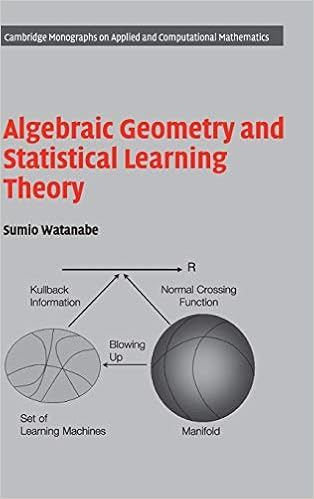## 31 janeiro 2020

### Geometria Algébrica e Teoria do Aprendizado Estatístico

Muito interessante este livro:

A parametric model in statistics or a learning machine in information science is called singular if the map from the parameter to the probability distribution is not one-to-one, or if its Fisher information matrix is not positive definite. A lot of statistical models are singular, for example, artificial neural networks, reduced rank regressions, normal mixtures, binomial mixtures, hidden Markov models, stochastic context-free grammars, Bayesian networks, and so on. In general, if a statistical model contains hierarchical structure, sub-module, or hidden variables, then it is singular.

If a statistical model is singular, then the log likelihood function can not be approximated by any quadratic form, resulting that the conventional statistical theory of regular statistical models does not hold. In fact, Cramer-Rao inequality has no meaning, asymptotic normality of the maximum likelihood estimator does not hold, and the Bayes a posteriori distribution can not be approximated by any normal distribution. Neither AIC corresponds to the asymptotic average generalization error nor BIC is equal to the asymptotic Bayes marginal likelihood. It has been difficult to study singular models, because there are so many types of singularities in their log likelihood functions.

[...]